Week 5 Day 1
Week 5 Day 1
Name *
ID Number
How are you today!
Q1 *
5 points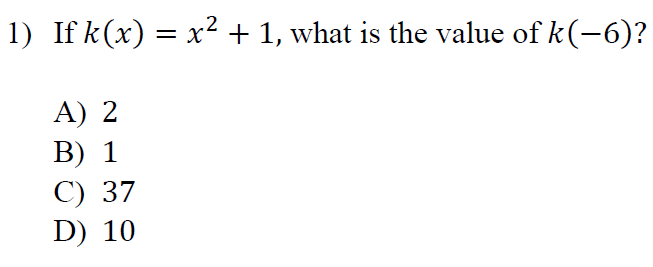Q2 *
5 points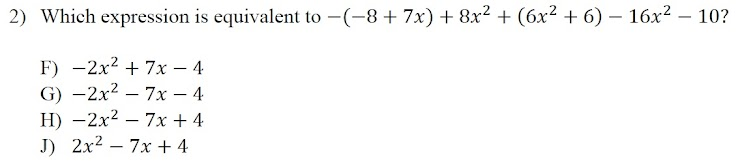Q3 *
5 points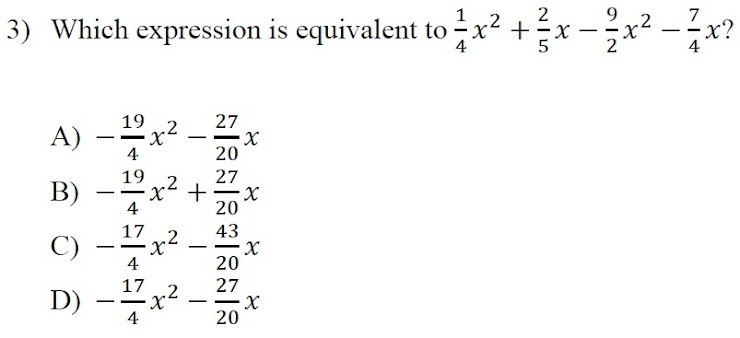Q 4
5 points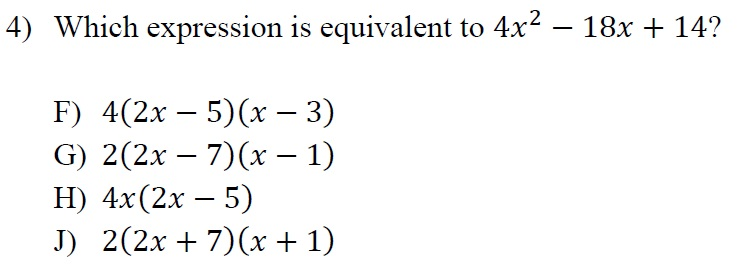Q 5
5 points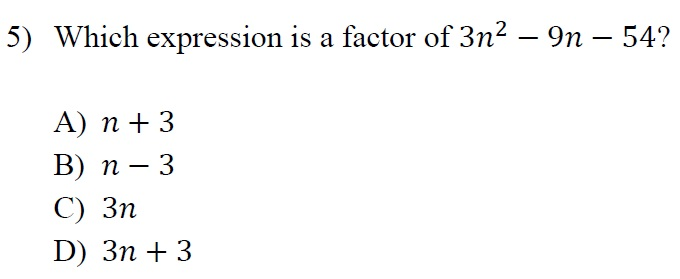Which inequalaity represent the phrase "At least " ? *
5 points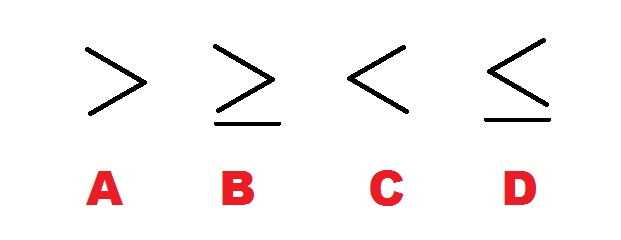Which inequalaity represent the phrase "No More Than " ? *
5 pointsWhich inequalaity represent the phrase "Fewer Than " ? *
5 pointsWhich inequalaity represent the phrase "Less Than " ? *
5 pointsIs this Relation represent a function , Yes or No ?
5 points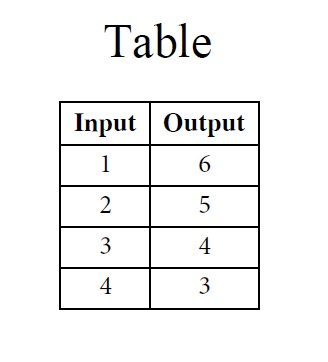Is this Relation represent a function , Yes or No ?
5 points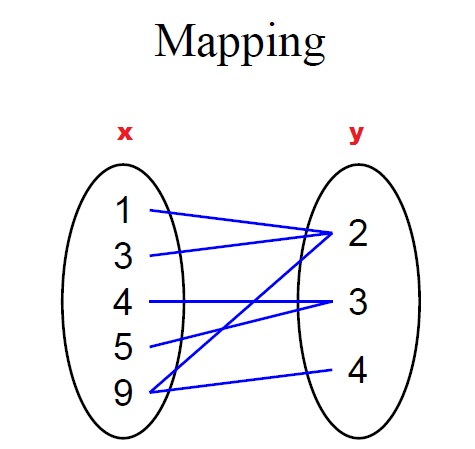Is this Relation represent a function , Yes or No ?
5 points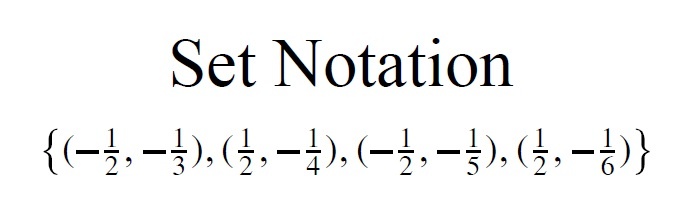What is the value of x?
5 points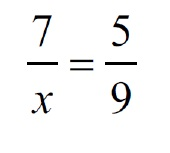What is the slope m of the line shown below?
5 points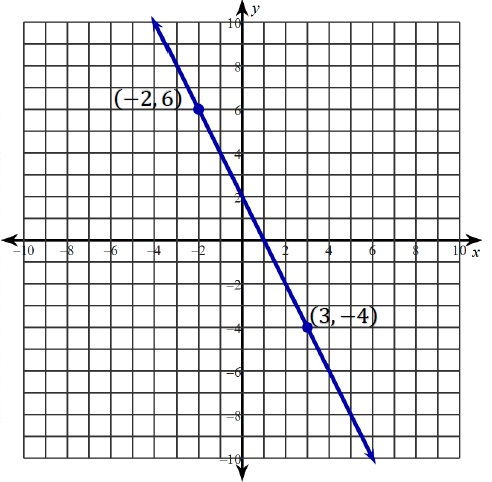What is the value of b of the line shown below?
5 pointsWhat is the zero of the line shown below?
5 pointsDetermine which of these represent the system of equations :
5 points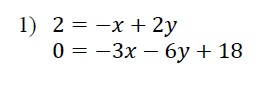Determine which of these represent the system of equations :
5 points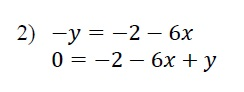Determine which of these represent the system of equations :
5 pointsWhich ordered pair is in the solution set of the inequality 7x+5y<20
0 points
Which letter on the graph represent the "Axis of Symmetry " ?
5 points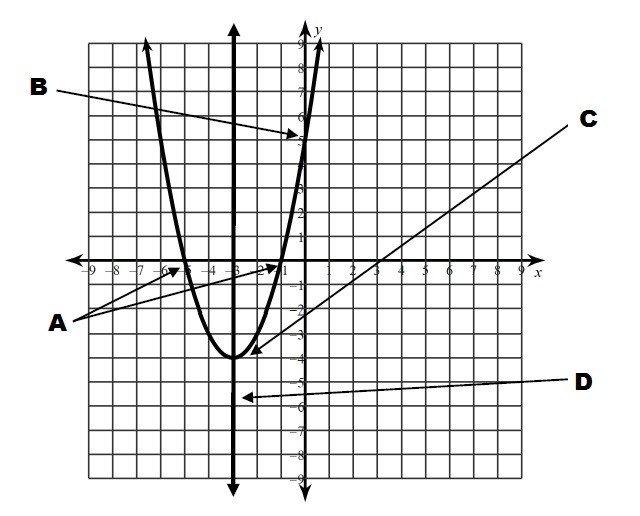Which letter on the graph represent the "solutions" ?
5 pointsWhich letter on the graph represent the "Vertex" ?
5 points